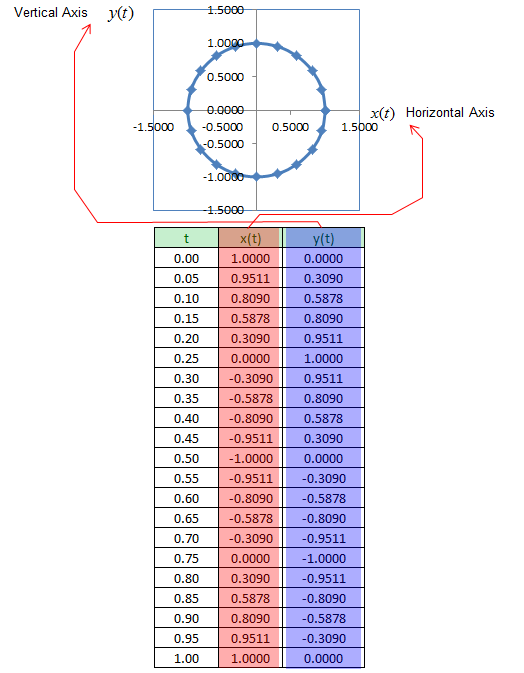Engineering Math - Quick Reference                                 Home : www.sharetechnote.com Parametric Function/Parametric Plot   Parametric Plot is a special type of techique to plot a data set. As you see in the illustration shown below, in ordinary graph the horizontal axis is mapped to the independent variable of a function and vertical axis is mapped to the dependent variable (i.e, the function value). But in Parametric Plot, both horizontal axis and vertical axis are independent variables (i.e, the function values). The two axis in Parametric plot is determined by an hidden independent variable called 'parameter'.   In engineering, this is one of the most frequently used form of graph. If you are new to this concept, I recommend you to read through this over and over until you clearly understand the concept.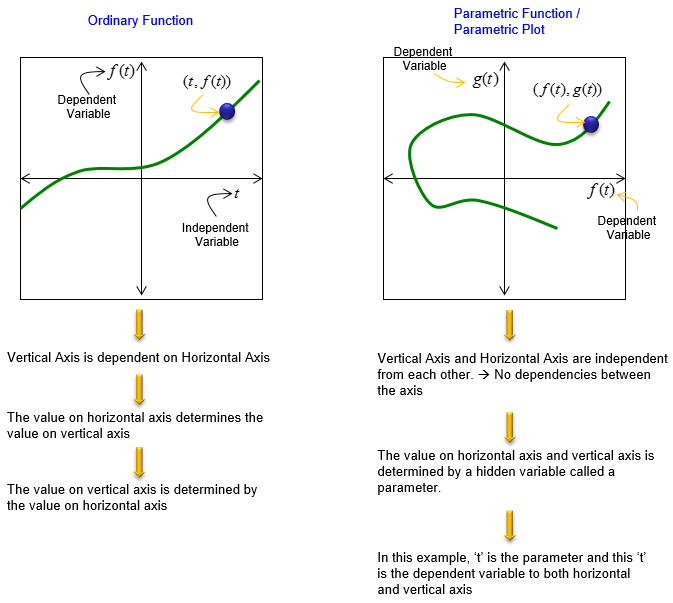It may not be so clear to you yet just by the explanation shown above. Let's take a look at a specific example.   Let's suppose we have a data set as shown below. In this example, 't' is independent variable and they are given. x(t) is determined by 't' based on the function cos(2 pi t). y(t) is determined by 't' based on the function sin(2 pi t).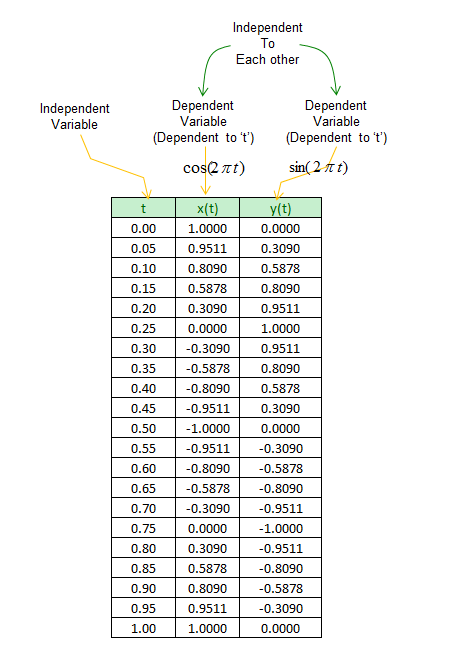Now let think of how to represent these data in Ordinary Graph. In order to represent x(t) and y(t) in ordinary graph, we need to draw two plots as shown below.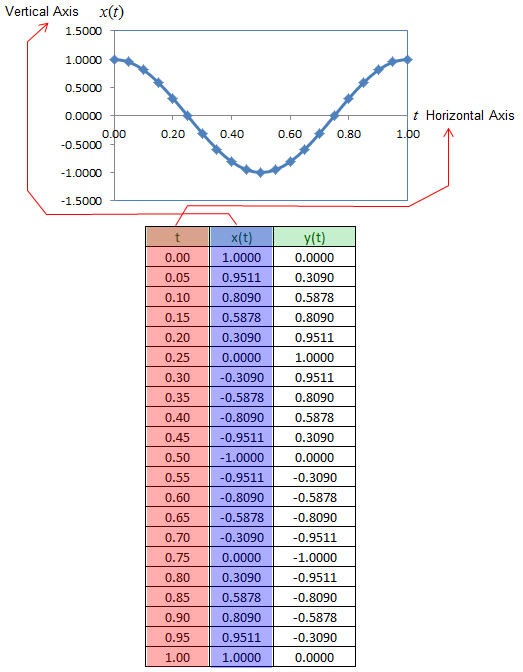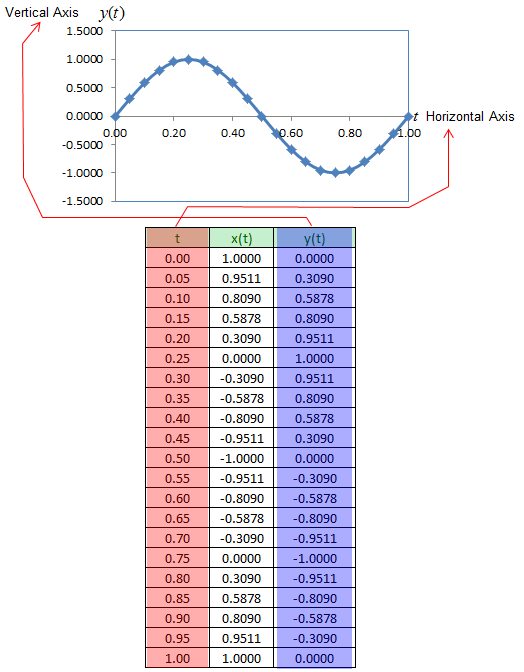If you represent this as a parametric plot, you can represent both x(t) and y(t) in a single graph as shown below. In this case, the independent variable 't' is not directly visible in the plot.